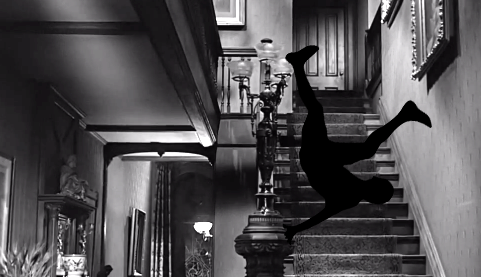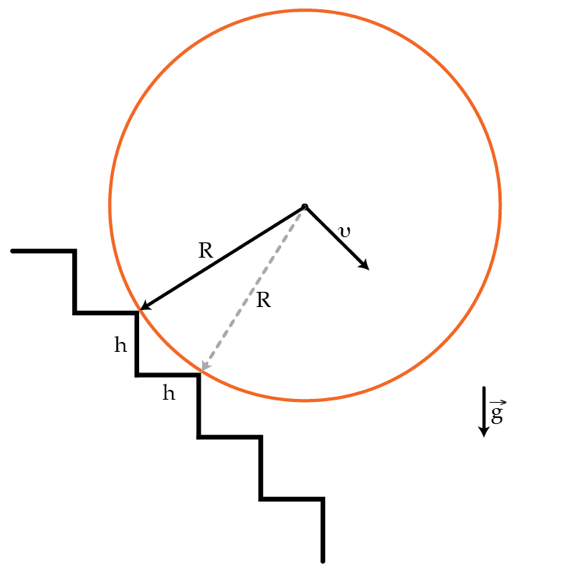# Rolling Down The StairsA solid homogeneous cylinder of mass $M=1 ~\mbox{kg}$ and radius $R=2~\mbox{m}$ rolls down the stairs as shown in the figure below. The height and the width of the steps are both $h=20~\mbox{cm}$. Assume that the collisions between the cylinder and the steps are inelastic and that the cylinder always remains in contact with the stairs. What will be the terminal velocity (on average) in m/s of the cylinder's center of mass?×

Problem Loading...

Note Loading...

Set Loading...